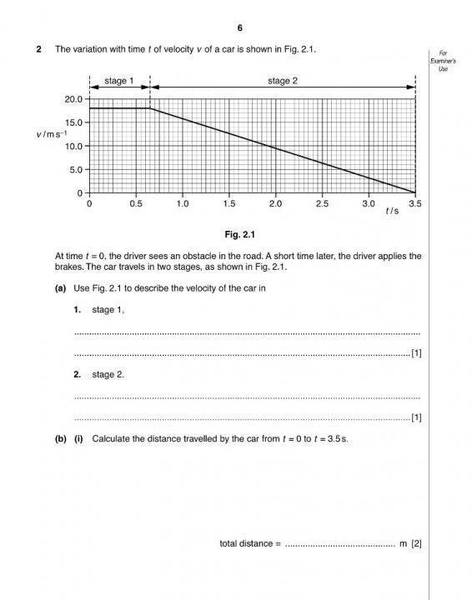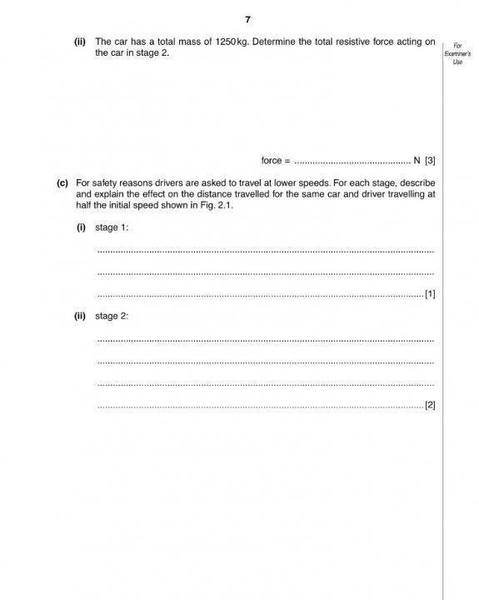# Kinematics car acceleration question

## Homework StatementFor part c ii) of this question, the new distance is now 1/4 of the previous distance as both acceleration are the same.

But why are both the acceleration the same?

v^2= u^2 +2as

## The Attempt at a Solution

I know that the method is by using the above equation, and taking v as 0, we get s = u^2/2a.
So when the initial speed is now halved, we get s' = (u/2)^2 /2a , and ∴ s' = u^2/8a , which is ¼ of the previous distance.

But why are the acceleration the same for both cases?

The deceleration (negative acceleration because it is slowing down) is the same in both cases because it is assumed that the same braking effort was used in both cases. Remember accel/decel is the rate of change of velocity, and in both cases the car is slowing down at the same rate, regardless of initial velocity.

The deceleration (negative acceleration because it is slowing down) is the same in both cases because it is assumed that the same braking effort was used in both cases. Remember accel/decel is the rate of change of velocity, and in both cases the car is slowing down at the same rate, regardless of initial velocity.

But how do we know that same braking effort is used in both cases? Is it because the resistive force are the same for both cases too?

haruspex
Homework Helper
Gold Member
2020 Award
But how do we know that same braking effort is used in both cases? Is it because the resistive force are the same for both cases too?
I agree with you - there is no sound basis for assuming the deceleration is the same. The driver, presumably, merely requires to stop before reaching the obstacle, but has no reason to stop well short of it. If the initial speed is lower, the driver may choose to decelerate more gently, and/or start braking later. It's a poorly specified question.
If I had to guess, I'd go for covering the same distance and starting to brake at the same point in time, but decelerating more gently.

I agree with you - there is no sound basis for assuming the deceleration is the same. The driver, presumably, merely requires to stop before reaching the obstacle, but has no reason to stop well short of it. If the initial speed is lower, the driver may choose to decelerate more gently, and/or start braking later. It's a poorly specified question.
If I had to guess, I'd go for covering the same distance and starting to brake at the same point in time, but decelerating more gently.

Yeah. Are the resistive force acting on the car at stage 2 the same for both cases? Since same car, same driver..? But if the car is moving at a slower speed, then shouldn't the counter forces (e.g. Air resistance) be lesser?
Because in determining the resistive force, we can know the deceleration of the car (whether they are the same )

haruspex